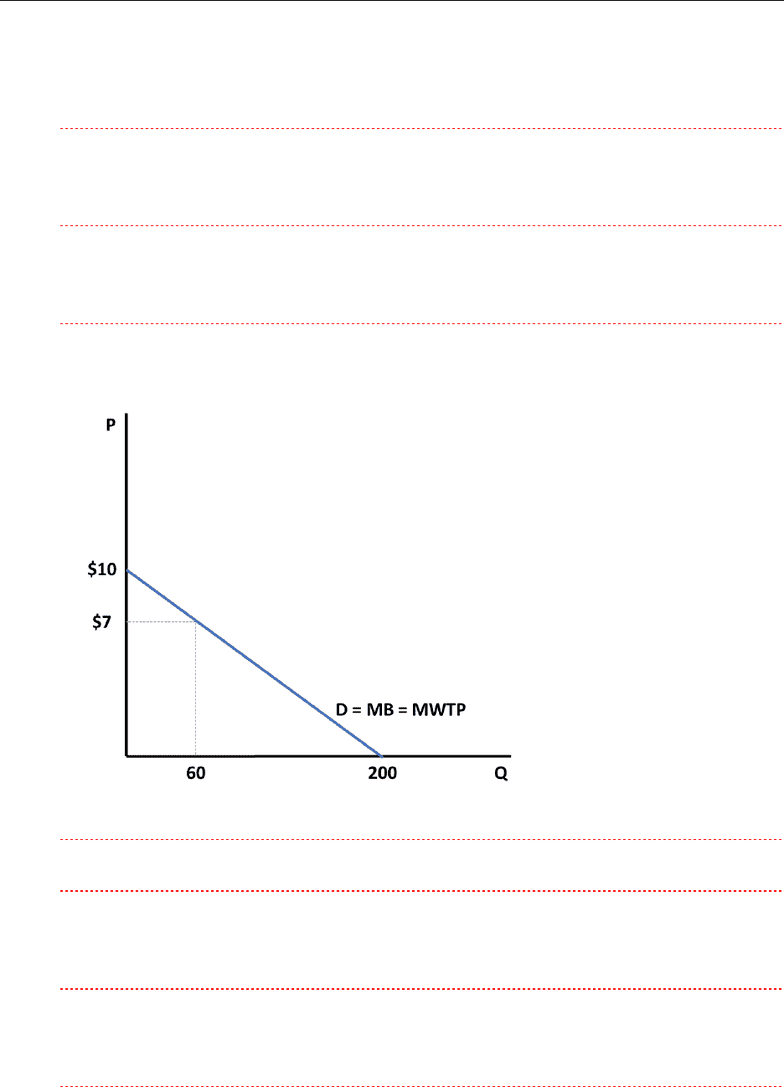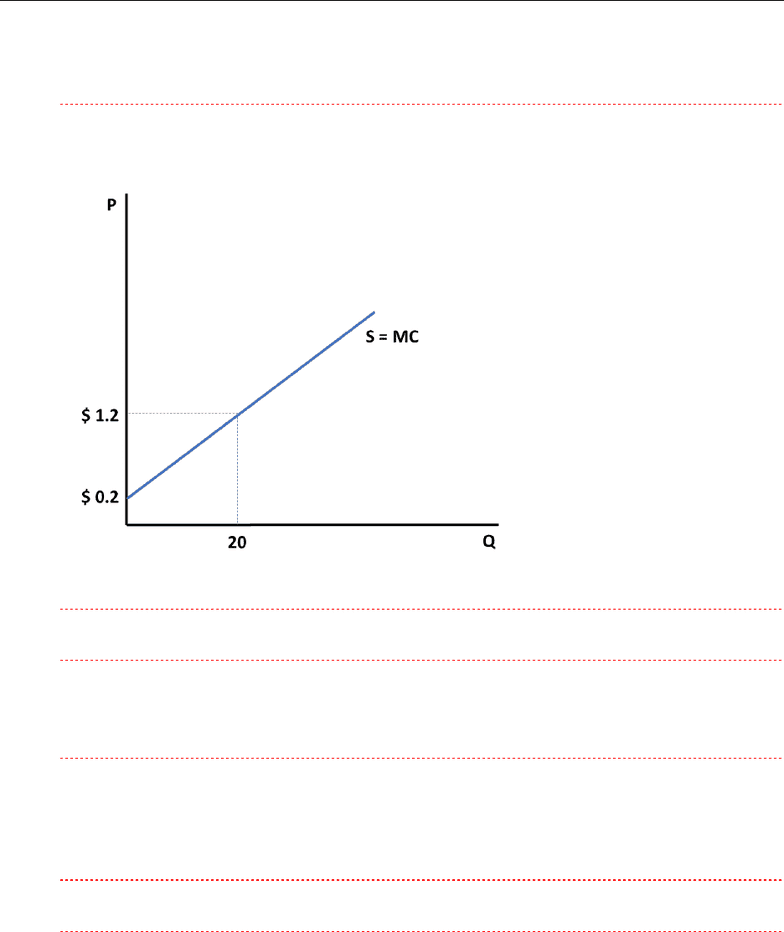Class Notes (1,100,000)
CA (620,000)
SFU (10,000)
ECON (500)
ECON 103 (100)
Lecture 5

# ECON 103 Lecture Notes - Lecture 5: Economic Surplus, Economic Equilibrium

Department
Economics
Course Code
ECON 103
Professor
Junjie Liu
Lecture
5

This preview shows page 1. to view the full 5 pages of the document.Econ 103 Tutorial #5 Week 8
1
1. Melissa buys an iPod for \$120 and gets consumer surplus of \$80.
a. What is her willingness to pay?
\$200
b. If she had bought the iPod on sale for \$90, what would her consumer surplus have been?
\$110
c. If the price of an iPod were \$250, what would her consumer surplus have been?
\$0
2. Amy has a demand function for apples given by Q = 200 20P.
a. Graph and calculate her MWTP (or MB) and TWTP (or TB) at a quantity of 60 apples.
MWTP = MB = \$7
TWTP = TB = ½ × 60 × \$ (10 + 7) = \$510
b. Calculate Amy’s consumer surplus (CS) if the price of apples is \$1.50.
CS = ½ × 170 × \$ (10 - \$1.5) = \$722.5
c. If the apples are free and Amy could have as many as she wants, what would be her CS?
CS = ½ × 200 × \$10 = \$1000
###### You're Reading a Preview

Unlock to view full version

Only page 1 are available for preview. Some parts have been intentionally blurred.Econ 103 Tutorial #5 Week 8
2
d. If the apples are free but the quantity is restricted to 100 apples, what would be her CS?
CS = ½ × 100 × \$ (10 + 5) = \$750
3. Ivy grows apples, and her supply function is given by Q = 20P 4.
a. Graph and calculate her MC and TC at a quantity of 20 apples.
MC = \$1.2
TC = ½ × 20 × \$ (0.2 + 1.2) = \$14
b. Calculate Ivy’s producer surplus (PS) if the price of apples is \$1.50.
PS = ½ × 26 × \$ (1.5 - 0.2) = \$16.9
c. If the price of apples remains at \$1.50, but Ivy’s MC of producing apples decreases by 20
percent. By how much will Ivy’s PS change?
New Supply Curve: MC = (1 0.20)×(0.2 +0.05Q) P = 0.16 + 0.04 Q
ΔPS = ½ × 33.5 × \$ (1.5 - 0.16) - ½ × 26 × \$ (1.5 - 0.2) = \$5.545
4. A friend of yours is considering two cell phone service providers. Provider A charges \$100
per month for the service regardless of the number of phone calls made. Provider B does not
have a fixed service fee but instead charges \$1 per minute for calls. Your friend’s monthly
demand for minutes of calling is given by the equation QD = 150 − 50P, where P is the price
of a minute.
a. With each provider, what is the cost to your friend of an extra minute on the phone?
###### You're Reading a Preview

Unlock to view full version